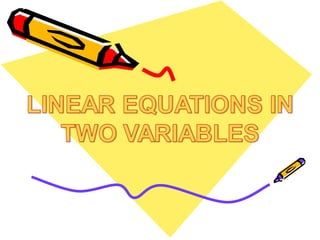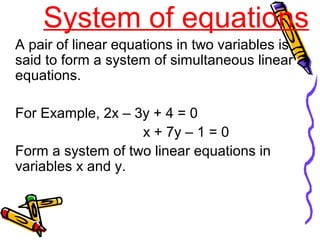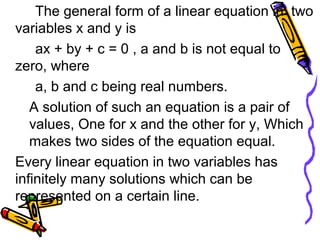Diese Präsentation wurde erfolgreich gemeldet.

# Maths ppt linear equations in two variables

Anzeige
Anzeige
Anzeige
Anzeige
Anzeige
Anzeige
Anzeige
Anzeige
Anzeige
Anzeige
Anzeige×

1 von 14 Anzeige

# Maths ppt linear equations in two variables

AN EQUATION WHICH CAN BE WRITTEN IN THE FORM OF ax+by+c=0 WHERE a,b and c ARE REAL NUMBERS.

YOU WILL GET TO KNOW HOW TO REPRESENT THE EQUATIONS IN A GRAPH.

AN EQUATION WHICH CAN BE WRITTEN IN THE FORM OF ax+by+c=0 WHERE a,b and c ARE REAL NUMBERS.

YOU WILL GET TO KNOW HOW TO REPRESENT THE EQUATIONS IN A GRAPH.

Anzeige
Anzeige

### Maths ppt linear equations in two variables

1. 1. System of equations A pair of linear equations in two variables is said to form a system of simultaneous linear equations. For Example, 2x – 3y + 4 = 0 x + 7y – 1 = 0 Form a system of two linear equations in variables x and y.
2. 2. The general form of a linear equation in two variables x and y is ax + by + c = 0 , a and b is not equal to zero, where a, b and c being real numbers. A solution of such an equation is a pair of values, One for x and the other for y, Which makes two sides of the equation equal. Every linear equation in two variables has infinitely many solutions which can be represented on a certain line.
3. 3. GRAPHICAL SOLUTIONS OF A LINEAR EQUATION Let us consider the following system of two simultaneous linear equations in two variable. 2x – y = -1 3x + 2y = 9 Here we assign any value to one of the two variables and then determine the value of the other variable from the given equation.
4. 4. For the equation 2x –y = -1 ---(1) 2x +1 = y Y = 2x + 1 3x + 2y = 9 --- (2) 2y = 9 – 3x Y = 9-3x X 0 2 Y 1 5 X 3 -1 Y 0 6
5. 5. XX Y Y (2,5) (-1,6) (0,3)(0,1) X= 1 Y=3
6. 6. Let us take an example x + 2y = -1 ------------------ (i) 2x – 3y = 12 -----------------(ii)
7. 7. SUBSTITUTION METHOD x + 2y = -1 x = -2y -1 ------- (iii) Substituting the value of x in equation (ii), we get 2x – 3y = 12 2 ( -2y – 1) – 3y = 12 - 4y – 2 – 3y = 12 - 7y = 14 , y = -2 ,
8. 8. SUBSTITUTION Putting the value of y in eq. (iii), we get x = - 2y -1 x = - 2 x (-2) – 1 = 4 – 1 = 3 Hence the solution of the equation is ( 3, - 2 )
9. 9. ELIMINATION METHOD • In this method, we eliminate one of the two variables to obtain an equation in one variable which can easily be solved. Putting the value of this variable in any of the given equations, The value of the other variable can be obtained. • For example: we want to solve, 3x + 2y = 11 2x + 3y = 4
10. 10. Let 3x + 2y = 11 --------- (i) 2x + 3y = 4 ---------(ii) Multiply 3 in equation (i) and 2 in equation (ii) and subtracting eq. iv from iii, we get 9x + 6y = 33 ------ (iii) 4x + 6y = 8 ------- (iv) 5x = 25 x = 5
11. 11. • putting the value of y in equation (ii) we get, 2x + 3y = 4 2 x 5 + 3y = 4 10 + 3y = 4 3y = 4 – 10 3y = - 6 y = - 2 Hence, x = 5 and y = -2